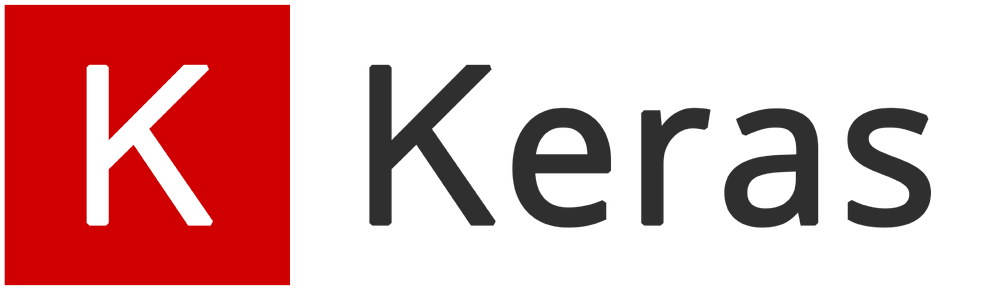# Here is how to use Keras to train an image classification model.使用keras訓練圖像分類

Source: Deep Learning on Medium`#線性堆疊模型，將之後神經網路層加入model = Sequential()#construck CNN Modelmodel.add(Conv2D(filter=32, (3, 3), input_shape=input_shape))model.add(Activation('relu'))model.add(MaxPooling2D(pool_size=(2, 2)))model.add(Conv2D(filter=32, (3, 3)))model.add(Activation('relu'))model.add(MaxPooling2D(pool_size=(2, 2)))model.add(Conv2D(filter=64, (6, 6)))model.add(Activation('relu'))model.add(MaxPooling2D(pool_size=(2, 2)))model.add(Dropout(0.5))model.add(Flatten())model.add(Dense(18)) #打上自己資料夾中有幾個分類model.add(Activation('softmax')) #多個的話一定要用Softmaxmodel.compile(loss='categorical_crossentropy', optimizer='rmsprop',metrics=['sparse'])`